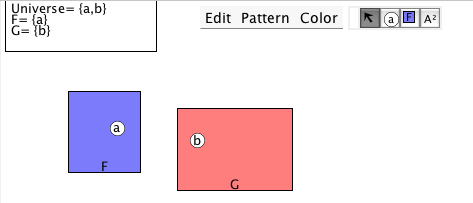# Tutorial 13: An Introduction to Truth in Predicate Logic

Logical System

### Skill to be acquired:

To learn how to interpret simple predicate logic formulas as being true or false.

### Why this is useful:

This helps in proving invalidity by the technique of displaying a counter-example.

### The Tutorial:

In propositional logic, we just took it that each of the atomic propositions either is true or is false-- we did not look into the structure of the propositions.

Truth can be discussed in more detail in predicate logic.

To do this we first need entities for the constant terms to refer to. So, for example, with the formula Gb we need something for the b to refer to. We imagine universes, scenarios or worlds each of which has a b in it; a useful way of depicting one universe with a b in it is just to draw a small circle labelled b.

We also need to be able to interpret the idea of an object in a universe possessing or lacking a property-- within a universe containing {a,b} the object a may have the property G and the object b may lack the property G. If the entities in a universe have been depicted by little circles with labels, a way of depicting whether an object has the property G is to draw a square labelled G around those objects that do.

## Exercises to accompany Predicate Tutorial 3

The Interpretation in Predex3 should show an Interpretation like thisIf it does not, just draw a similar one. [Sometimes, re-loading the page will cause the diagrams to draw.]

### Exercise 2 (of 3)

Exercise 3 (of 3)

When we are trying to establish that an argument is invalid, we look for a counter-example to it. A counter-example is a world or scenario in which all the premises are true and the conclusion false.

For example, consider the argument

If Bert is famous or Arthur is a gambler, then Arthur is famous and Bert is a gambler
Bert is a gambler.
Arthur is famous.

Therefore
Bert is famous or Arthur is a gambler.

which symbolizes to

(Fb∨Ga)⊃(Fa∧Gb)

Gb

Fa

Fb∨Ga

In the previouslly drawn world all the premises are true and the conclusion false-- the depicted world constitutes a counter-example to the argument. It proves that the argument is invalid.

We can also use a device that we used in propositional logic. Instead of trying to see if all the premises can be true and the conclusion false at one and the same time, we can make a list of all the premises and the negation of the conclusion. Thus

(Fb∨Ga)⊃(Fa∧Gb), Gb, Fa, ~(Fb∨Ga)

and this entire list of formulas is true. In the jargon, the set of formulas is 'satisfiable'.

In practical cases you will be given the argument and have to produce the counter-example drawing yourself.

The program can do this for you.

If you decide to use the web application for the exercises you can launch it from here Deriver [Gentzen] — username 'logic' password 'logic'. Then either copy and paste the above formulas into the Journal or use the Deriver File Menu to Open Web Page with this address https://softoption.us/test/easyDeriver/CombinedExercisesEasyDGentzen.html .

Preferences

You may need to set some Preferences for this.

• you can check that the parser is set to gentzen.